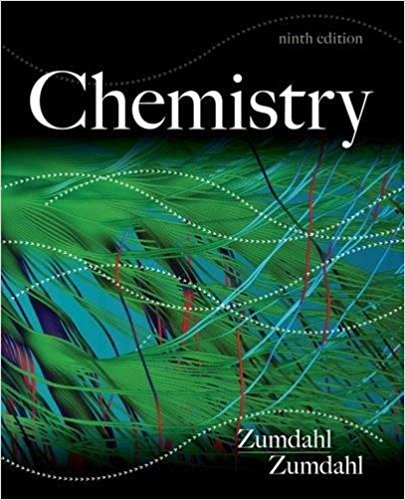×

×

# Solutions for Chapter 10: Liquids and Solids## Full solutions for Chemistry | 9th Edition

ISBN: 9781133611097Solutions for Chapter 10: Liquids and Solids

Solutions for Chapter 10
4 5 0 249 Reviews
30
5
##### ISBN: 9781133611097

Since 170 problems in chapter 10: Liquids and Solids have been answered, more than 91307 students have viewed full step-by-step solutions from this chapter. Chemistry was written by and is associated to the ISBN: 9781133611097. This textbook survival guide was created for the textbook: Chemistry, edition: 9. This expansive textbook survival guide covers the following chapters and their solutions. Chapter 10: Liquids and Solids includes 170 full step-by-step solutions.

Key Chemistry Terms and definitions covered in this textbook
• actinide series.

Elements that have incompletely filled 5f subshells or readily give rise to cations that have incompletely filled 5f subshells. (7.9)

• activity series

A list of metals in order of decreasing ease of oxidation. (Section 4.4)

• back-side attack

In SN2 reactions, the side opposite the leaving group, which is where the nucleophile attacks.

• basic anhydride (basic oxide)

An oxide that forms a base when added to water; soluble metal oxides are basic anhydrides. (Section 22.5)

In 13C NMR spectroscopy, a technique in which all 13C!1H splitting is suppressed with the use of two rf transmitters.

• chemical equation

A representation of a chemical reaction using the chemical formulas of the reactants and products; a balanced chemical equation contains equal numbers of atoms of each element on both sides of the equation. (Section 3.1)

• debye (D)

A unit of measure fordipole moments, where 1 debye = 10-18 esu×cm.

• dienophile

A compound that reacts with a diene in a Diels-Alder reaction.

• exothermic

Any process with a negative DH (the system gives energy to the surroundings).

• haloform reaction

A reaction in which a methyl ketone is converted into a carboxylic acid upon treatment with excess base and excess halogen, followed by aqueous acid.

• Homolytic bond cleavage

Cleavage of a bond so that each fragment retains one electron; formation of radicals.

• isoelectric point (pI)

For an amino acid, the specific pH at which the concentration of the zwitterionic form reaches its maximum value.

• joule (J)

The SI unit of energy, 1 kg@m2 >s2. A related unit is the calorie: 4.184 J = 1 cal. (Section 5.1)

• mass defect

The difference between the mass of a nucleus and the total masses of the individual nucleons that it contains. (Section 21.6)

• nonbonding pair

In a Lewis structure a pair of electrons assigned completely to one atom; also called a lone pair. (Section 9.2)

• pyrometallurgy

A process in which heat converts a mineral in an ore from one chemical form to another and eventually to the free metal. (Section 23.2)

• R (Section 3.3)

From the Latin, rectus, straight, correct; used in the R,S convention to show that the order of priority of groups on a chiral center is clockwise.

• racemic mixture

A mixture of equal amounts of the dextrorotatory and levorotatory forms of a chiral molecule. A racemic mixture will not rotate the plane of polarized light. (Section 23.4)

• Sanger dideoxy method

A method developed by Frederick Sanger for sequencing DNA molecules

• unimolecular

For mechanisms, astep that involves only one chemical entity.